Updated by Timothy Williams on Jul 08, 2014
REPORTTimothy Williams
Owner
27 items   1 followers   0 votes   5 views

# Goose Creek CISD - High School - Mathematics

1

## Converter Plus [free]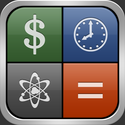Converter Plus is a unit converter with the most extensive list of currencies and units in hundreds of categories. Converter Plus also comes with many calculators like mortgage, loan, tip, fuel consumption and many others for everyday problems.

Exchange rates for all 167 world's currencies is refreshed automatically every 6 hours or on request.

2

## Math Ref Free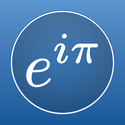Math Ref Free is a free version of the award winning education app Math Ref. This app gives you just a sample (over 700) of the over 1,400 helpful formulas, figures, tips, and examples that are included in the full version of Math Ref. So, if you're a student, teacher, or need math for work and want to try before you buy, this app is for you.

3

## iFormulas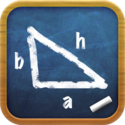IFormulas provides an clean slate to effortlessly look up mathematical formulas.

Categories :
Algebra, Calculus, Chemistry, Geometry, Electrical, Trigonometry, Physics

Features:
Hundreds of formulas
Saves Tab Bar Rearrangements
Favorite Formulas
Scrollable and Zoomable Formula View

Suggestions are welcomed. Please shoot me a message if you find a mistake.

4

## Algebra ChampNew to algebra? Still find that "x" a little intimidating? Download this free application and you'll be a champ in no time! Algebra Champ provides introductory level algebra skills practice with timed rounds, high scores, and a cage fight theme.

Designed for grades 6 - 8, Algebra Champ provides practice solving straightforward, single variable linear equations in an entertaining, game-like environment. Questions are randomly generated and presented in rounds of five. Answers are manageable integers (-10 to 10) and presented as multiple choice.

Beginners may need to jot down a step or two on a piece of paper. More advanced players will be able to solve these problems on the fly. Play a few rounds each day and you'll quickly gain an increased comfort level with entry level algebraic expressions, negative integers, and quick mental calculations.

5

## Exponents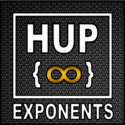This apps is designed to allow students to learn about the rules of exponents. The purpose is that this app will be a resource in the learning of exponent rules.

6

## HMH FUSE: Algebra 1WHAT
The award-winning and revolutionary HMH Fuse™ is a highly interactive and engaging curriculum designed for the Apple iPad. HMH Fuse Algebra 1 gives every student a personalized learning experience. Students and Teachers use video tutorials, StepReveal- the award winning way we guide students through step-by-step examples, homework help, quizzes, tips, hints, and many other integrated features to teach, review, and assess all concepts.

7

## Algebra Pro - Complete Workbook with ShowMe Lessons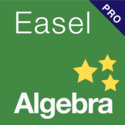The good news: the app is now free.
The entire workbook breaks down into the following sections:

The Language of Algebra - 8 questions
Real Numbers - 4 questions
Solving Linear Equations - 8 questions
Graphing Relations and Functions - 8 questions
Analyzing Linear Equations - 8 questions
Solving Linear Inequalities - 4 questions
Solving Systems of Linear Equations and Inequalities - 8 questions
Properties of Exponents - 8 questions
Polynomials - 8 questions
Factoring - 8 questions
Exponential and Radical Functions - 8 questions
Rational Functions and Equations - 8 questions
Statistics - 4 questions
Probability - 4 questions

8

## Video Calculus“The best Calculus lessons this reviewer has seen anywhere” — L. McMullin, The College Board.

Video Calculus covers everything from limits and derivatives to differential equations and vector calculus. By downloading Video Calculus you will have access to over 15 Featured Lessons for FREE and you’ll be able to preview any of the 250+ different lessons included in the app. With this app you can:
* Watch over 2 hours of select Calculus content for FREE from the “Featured Lessons” area
* Type and save your own notes for each lecture
* View full-color, illustrated lesson summaries for every lecture
* Learn Calculus from the world’s best math professor

9

## SAT Math Review : Free Edition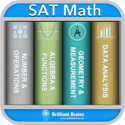Most comprehensive SAT Math App with over 1400 questions with solutions and 140 revision notes covering all math subjects which include:

1. Algebra & Functions
2. Geometry & Measurement
3. Data Analysis, Statistics and Probability
4. Number & Operations

Prepare to achieve the SAT scores you need to get into your top choice schools.

10

## Pocket AlgebraFeatured in the App Store's "New and Noteworthy" section for education!

Need some help in algebra? Then this app is right for you. This app will swiftly solve your math homework with ease, showing you all the steps along the way. Don't worry about having to have an internet connection because this app doesn't require one.

The app will help with the following topics:

• Calculate fractions
• Simplify fractions
• Complete the square
• Simplify square roots
• Find the factors of any integer
• Find the prime factorization of any integer

• SMS
• Email
11The fastest way to solve quadratic equations!

Quadratic+ solves quadratic equations with real and imaginary roots. It calculates the answer instantly as you type and is accurate to five decimal places. It's perfect for college and high school students taking algebra, calculus, or any math or science class.

12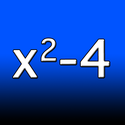This is a simple application that will factor quadratic equations, if possible. It's quick, handy, and easy.

Example: 6x² + 13x + 6 = (2x+3)(3x+2).

If you need to solve quadratics as well as factor them, you should check out iFactor and Solve Quadratics in the app store. It can handle any quadratic, including ones with complex roots.

13

## Equation Solver FREEWelcome to Equation Solver.

14

## Formulus Free - Formulas for Calculus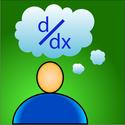Formulus Free is the perfect study tool. It is a simple, easy to use, easy to navigate collection of the most important formulas and topics for high school and college students taking Calculus and Differential Equations.

Includes the following subject areas:
Calculus
- Algebra & Geometry
- Derivatives
- Graphing
- Hyperbolics
- Integrals
- Limits & Continuity
- Matrices
- Series & Sequences
- Trigonometry
- Vectors
Differential Equations
- 1st Order Linear Equations
- 2nd Order Linear Equations
- Laplace Transforms

Support for Linear Algebra equations COMING SOON!!!

15

## MathwaySolve your math problems anywhere, anytime.

Bring the solving power of Mathway to your iOS device, no network access required! Get free instant answers to your math problems, or subscribe to include step-by-step work and explanations. With hundreds of millions of problems already solved, Mathway is the #1 problem solving resource available for students, parents, and teachers.

Mathway solves problems in Basic Math, Pre-Algebra, Algebra, Trigonometry, Precalculus, Calculus, Statistics, Finite Math, Linear Algebra, and Chemistry.

16

## Free Graphing Calculator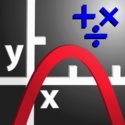1) Scientific Calculator. Simple to grasp and easy to use, but powerful features are available when you need them. Available functions include the following:

• the usual arithmetic functions and exponentiation.
• square root, cube root, nth root, natural log, log base 10, log of arbitrary base, absolute value, factorial, permutations (nPr), combinations (nCr), modulus, random integer, bell curve, cumulative normal distribution, decimal to fraction.

2) Graphing. Capabilities:

• Graph up to four equations at once.
• Graphs are labeled.
• You can drag the graph or pinch to zoom in or out.
• Calculator can find roots and intersections.
• Graph in polar coordinates.
• Graph parametric equations

17

## Quick Graph: Your Scientific Graphing Calculator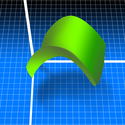Quick Graph is the best graphic calculator available on the AppStore!

It is a powerful, high quality, graphic calculator that takes full advantage of the multitouch display and the powerful graphic capabilities of the iPad and iPhone, both in 2D and 3D. A simple, yet intuitive interface that makes it easy to enter and/or edit equations and visualize them in mathematical notation.
It's capable of displaying explicit and implicit (opt) equations as well as inequalities (opt) in both 2D and 3D, in all standard coordinate systems: cartesian, polar, spherical and cylindrical, all with amazing speed and beautiful results, which can be copied, emailed or saved to the photo library.

18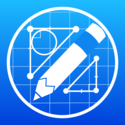With Geometry Pad you can create fundamental geometric shapes, explore and change their properties, and calculate metrics. The shapes are displayed on a scrollable and zoomable workbook with a rectangular coordinate system.

19

## Mathematical FormulasMathematical Formulas is the perfect app for you who likes mathematics and easily forgets formulas which you need in certain situation. Without a good app, it's tough to remember all these complicated and easy forgettable formulas. You don't even want to write down all you formulas on ten or even 20 different post-it papers and loosing them all the time. But hey, why don't have them in ONE place and easily access them whenever you want to?
Besides the application support TV-out so you can easily show the formulas for other not only yourself!

This app is especially made for this kind of a situations. Mathematical Formulas contains all the basic functions you'll need. You won't no more worry about forgetting the basic formulas such as the quadratic formula and etc.

20

## Pi Cubed Lite
Pi Cubed is a visual math application designed from the ground up for a touch-based interface. Unlike traditional calculators, Pi Cubed lets you construct mathematical expressions, using an interactive menu system, which are then typeset and evaluated instantly. The use of the entire screen, combined with the capability to lay out equations as they would be written on a piece of paper, makes even complex calculations easy to follow. Common touch gestures, like pinch-zooming and touch-based panning, enhance the entry and editing process.

A diverse range of mathematical operations are supported, including logarithms, trigonometric functions, arbitrary roots, exponents, and factorials.

21

## iformula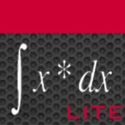THE ORIGINAL

NEW !!! Awarded with the iPhone apps plus „Hottest“ award from iphoneappsplus for outstanding education apps !!!

This is the iformula lite free version, please also have a look at the iformula pro version, which includes much more math formula, equation topics and other functions, like scientific calculator, calc links, graph, notes, printing, send equations to social networks or email them and much more at iTunes store !

iformula is a math formula, equation library, which was specially developed for academic students, engineers, high school students with math courses and scientist with higher mathematics skills.

Do you also sometimes forget equations, formulas you have learned very hard years before at school, university or somewhere else ? Now you need them again ? The solution is called iformula !

22

## Graphing Calculator by Desmos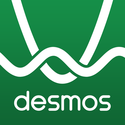Explore math with Desmos! Graph functions, create function tables, explore transformations, plot points, animate your graphs, and more – for free!

At Desmos, we imagine a world of universal math literacy and envision a world where math is accessible and enjoyable for all students. We believe the key is learning by doing.

To achieve this vision, we’ve started by building the next generation of the graphing calculator. Using our powerful and blazingly-fast math engine, the calculator can instantly plot any equation, from lines and parabolas up through derivatives and Fourier series. Sliders make it a breeze to demonstrate function transformations. It's intuitive, beautiful math. And best of all: it's completely free.

Features:

Graphing: Plot polar, cartesian, or parametric graphs. There’s no limit to how many expressions you can graph at one time - and you don’t even need to enter expressions in y= form!

23

## The Official SAT Question of the Day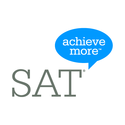Can you answer today's SAT question? Tease your brain with real SAT questions and SAT preparation materials from the test maker.

With the question of the day application you can answer a real SAT question each day.

Note: The SAT question of the day application works in portrait mode only.

24

## IntelliVocab for SATIntelliVocab personalizes the English vocabulary learning for competitive exams like SAT. This app is built by students of MIT based on the latest research in Artificial Intelligence, Machine Learning and Web Semantics.
It uses latest machine learning algorithms and web semantic techniques to collapse billions of possibilities into comprehensible probabilistic profile information, in real-time. It completely controls the learning environment making you free from the trouble of forming your own learning approach. All you need to do is interact. The more you practice, the more it learns about you and figures out words that are difficult for you. It ensures you achieve top score in SAT.

25

## AP Exam Prep - Calculus AB/BC, Chemistry, English Language, English Literature, Environmental Science, Human Geograph...Want to know what it feels like to have the world at your fingertips? Using this app, you just might get a glimmer. With content derived directly from the successful McGraw-Hill AP 5 Steps to a 5 series, the questions and detailed explanations closely mirror the topic, format, and degree of difficulty found in the actual AP exams. So don't leave anything to chance. Optimize your study time today with AP Exam Prep, the most comprehensive AP app on the market.